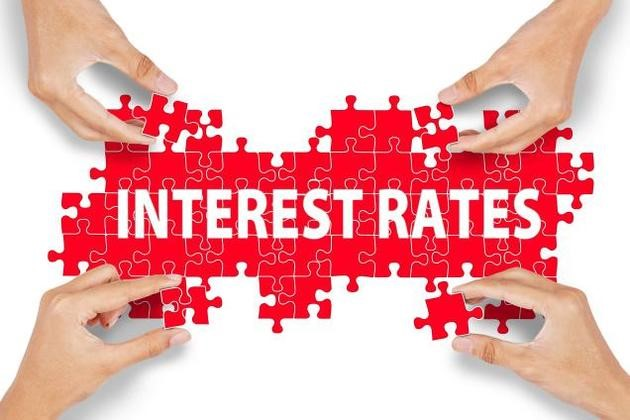# Interest Rates in Practice of the Banks

on February 1, 2017There are many methods, usually banks use to calculate the interest rates and each method will change the amount of interest that you have to pay. If you know that how to calculate the interest rates, you will better understand your loan contract with your bank. In addition, you will be in a better position to negotiate your interest rate with your bank.

Bank loans carry two types of interest rates, the stated or nominal interest rate and the effective interest rate or annual percentage rate (APR).

Stated Rates

The stated interest rate is just what it says. It is the simple interest rate that the bank gives you as the interest rate on the loan. This interest rate does not take the effect of compound interest into account.

Annual Percentage Rate

The annual percentage rate (APR) is the actual amount you pay to borrow the money or the rent on the money you have borrow. The APR, also called the effective interest rate, takes the effect of compound interest into account as well.

When a bank quotes you an interest rate, it’s quoting what’s called the effective rate of interest, also known as the annual percentage rate (APR). The APR or effective rate of interest is different than the stated rate of interest, due to the effects of compounding of interest.

Which Rate is Higher?

The APR is obviously higher than the stated interest rate unless compound interest is not involved. If you take out a simple interest loan and pay the entire loan off at the end of some time period, then the APR and stated rate will be the same.

However, if you take out an installment loan, for example, the APR is considerably higher than the stated interest rate.

How to Calculate Interest on a One-Year Loan?

If you borrow \$1,000 from a bank for one year and have to pay an agreed \$60 in interest for that year, your stated interest rate is 6%.

Here is the calculation:

Effective Rate on a Simple Interest Loan = Interest/Principal

= \$60/\$1000 = 6%

Your annual percentage rate or APR is the same as the stated rate in this example because there is no compound interest to consider. This is a simple interest loan.

Meanwhile, this particular loan becomes less favorable if you keep the money for a shorter period of time. For example, if you borrow \$1,000 from a bank for 120 days and the interest rate remains at 6%, the effective annual interest rate is much higher.

Effective rate = Interest/Principal X Days in the Year (360)/Days Loan is Outstanding

Effective rate on a Loan with a Term of Less Than One Year

= \$60/\$1000 X 360/120 = 18%

The effective rate of interest is 18% since you only have use of the funds for 120 days instead of 360 days.

Interest Rates in Practice of the Banks

### Related Posts

Take a look at these posts